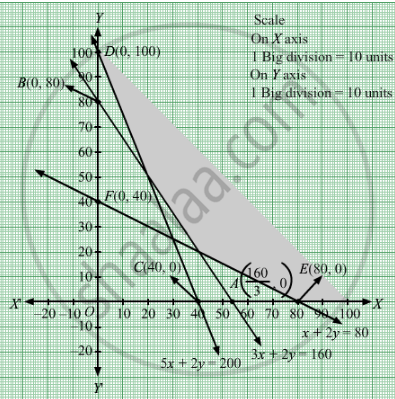Department of Pre-University Education, KarnatakaPUC Karnataka Science Class 12

# The Maximum Value of Z = 4x + 3y Subjected to the Constraints 3x + 2y ≥ 160, 5x + 2y ≥ 200, X + 2y ≥ 80; X, Y ≥ 0 is (A) 320 (B) 300 (C) 230 (D) None of These - Mathematics

MCQ

The maximum value of Z = 4x + 3y subjected to the constraints 3x + 2y ≥ 160, 5x + 2y ≥ 200, x + 2y ≥ 80; xy ≥ 0 is

#### Options

• 320

•  300

• 230

•  none of these

#### Solution

none of these

We need to maximize the function Z = 4x + 3y
Converting the given inequations into equations, we obtain

$3x + 2y = 160, 5x + 2y = 200, x + 2y = 80, x = 0 \text{ and } y = 0$

Region represented by 3x + 2y ≥ 160:
The line 3x + 2y = 160  meets the coordinate axes at $A\left( \frac{160}{3}, 0 \right)$ and B(0, 80) respectively. By joining these points we obtain the line 3x + 2y = 160.Clearly (0,0) does not satisfies the inequation 3x + 2y ≥ 160. So,the region in xy plane which does not contain the origin represents the solution set of the inequation 3x + 2y ≥ 160.

Region represented by 5x +2y ≥ 200 :
The line 5x +2y = 200 meets the coordinate axes at C(40,0) and D(0, 100) respectively. By joining these points we obtain the line 5x +2y = 200.Clearly (0,0) does not satisfies the inequation 5x +2y ≥ 200. So,the region which does not contain the origin represents the solution set of the inequation 5x +2y ≥ 200.

Region represented by x +2y ≥ 80:
The line x +2y = 80 meets the coordinate axes at E(80,0) and F(0, 40) respectively. By joining these points we obtain the line x +2y = 80.Clearly (0,0) does not satisfies the inequation x +2y ≥ 80. So,the region which does not contain the origin represents the solution set of the inequation x+2y ≥ 80.

Region represented by x ≥ 0 and y ≥ 0:
Since, every point in the first quadrant satisfies these inequations. So, the first quadrant is the region represented by the inequations x ≥ 0, and ≥ 0.
The feasible region determined by the system of constraints 3x + 2y ≥ 160, 5x +2y ≥ 200, x +2y ≥ 80, x ≥ 0, and y ≥ 0 are as follows.Here, we see that the feasible region is unbounded. Therefore,maximum value is infinity.

Concept: Introduction of Linear Programming
Is there an error in this question or solution?

#### APPEARS IN

RD Sharma Class 12 Maths
Chapter 30 Linear programming
MCQ | Q 7 | Page 67